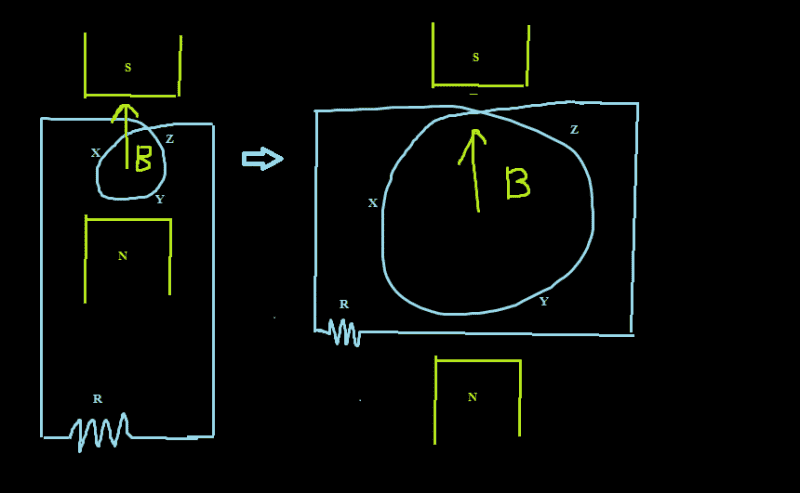# Problem on induced EMF

• arpon

## Homework Statement[/B]
Suppose, there is a 'constant' magnetic field ##B## in the upward direction. A loop of conductive wire(XYZ in the picture, which is connected to a closed circuit with resistance R) is placed horizontally on it. The area of the loop is being increased with time. Will there be any current flow in the circuit?

## Homework Equations

##\mathcal {E} = -\frac{d \phi}{dt}##
##\phi = BA cos \theta##

## The Attempt at a Solution

I think, as the area is changing, there will be a current flow proportional to the rate of change of area inclosed by the loop.

It's good to think of this induced emf as a consequence of the Lorentz force on the charge carriers in the wire. For the loop the velocity of the wire segments is outwards, perpendicular to B. The Lorentz force is ##\propto \vec v \times \vec B##, so clockwise.

The xyz directions require some explanation...
And ## \Delta \Phi## due to moving the outer loop into the B field (and changing its area as well ?) is a complication I disregarded.

And ΔΦ \Delta \Phi due to moving the outer loop into the B field (and changing its area as well ?) is a complication I disregarded.
Can't I calculate the induced EMF in this way? :
##\mathcal E = \frac{d\Phi}{dt} = \frac{d(BA)}{dt} = B \frac{dA}{dt}##
The Lorentz force is ∝v⃗ ×B⃗ \propto \vec v \times \vec B, so clockwise.
And how can I calculate the induced EMF or current flow by the formula you mentioned: ##\vec F = q \vec v \times \vec B## [##q## is the total charge of the charge carriers in the wire]
The xyz directions require some explanation...
What kind of explanation?

Can't I calculate the induced EMF in this way? :
##\mathcal E = \frac{d\Phi}{dt} = \frac{d(BA)}{dt} = B \frac{dA}{dt}##
Yes you can. dA is for the entire loop, not just the circle part.

And how can I calculate the induced EMF or current flow by the formula you mentioned: ##\vec F = q \vec v \times \vec B## [##q## is the total charge of the charge carriers in the wire]
Hard work. It's done for you here . Wire is neutral, of course. But there are mobile charge carriers and immobile ones. You refer to the former, of course.

What kind of explanation?
A little better than just writing x, y and z around the circular loop. You do indicate that B is in the z direction. Add that the circular loop is in the xy plane. (And you can avoid the complication I mentioned by placing the rectangular part of the loop in the yz plane, so that that part of ##\Phi=0##

A little better than just writing x, y and z around the circular loop. You do indicate that B is in the z direction. Add that the circular loop is in the xy plane. (And you can avoid the complication I mentioned by placing the rectangular part of the loop in the yz plane, so that that part of Φ=0
Actuallly, I named the points on the circular loop as x,y,z ; I realize it was not wise, because it creates confusion with the x,y,z-axes. However, I am explaining it again, the magnetic field is in the Z direction. The rectangular part of the circuit is on the yz-plane, and the circular part(which is changing the area enclosed by itself) is on the xy-plane, i.e., perpendicular to the direction of magnetic field.
Now, I would request you to revise your posts keeping in mind this explanation.

As far as I can distinguish the way you intended the configuration is indeed the way I understood it. So: yes, the movement of the wire increasing the diameter of the loop induces an electromotive force. And it can be calculated using Faraday's law $$\mathcal E = \oint_{\partial \Sigma} \vec E \cdot d\vec \ell= -{d\over dt}\;\int_\Sigma \vec B \cdot \vec{dA}$$which comes down to ##B\; \frac{dA}{dt}##

•arpon